# Contents

Bernd Klaus and Wolfgang Huber , based on material by Michael Love  and Simon Anders ,

 Department of Biostatistics, Dana-Farber Cancer Institute and Harvard School of Public Health, Boston, US;

 European Molecular Biology Laboratory (EMBL), Heidelberg, Germany.

LAST UPDATE AT

Wed Oct 19 11:09:15 2016

# 1 Preparations

We first set global chunk options and load the neccessary packages.

library(BiocStyle)
library(rmarkdown)
library(geneplotter)
library(ggplot2)
library(plyr)
library(LSD)
library(DESeq2)
library(gplots)
library(RColorBrewer)
library(stringr)
library(topGO)
library(genefilter)
library(biomaRt)
library(dplyr)
library(EDASeq)
library(fdrtool)
library(org.Mm.eg.db)

data.dir <- file.path("/g/huber/users/klaus/Data/NCBI")

In this document we introduce a workflow for a typical RNA–Seq data analysis. We start from a “count table”, which summarizes the number of sequence reads mapping to each of the genes and discuss quality control, differential expression and enrichment analysis of the data. Important aspects of the R language and Bioconductor data structures for high–dimensional genomic data are discussed along the way.

We re—analyze RNA–Seq data obtained by comparing the expression profiles of WT mice to mice harboring a deletion that is associated with a MYC enhancer which is required for proper lip/palate formation. The data was published along with the following paper:

Note that in the workflows below, we do not aim at giving a comprehensive treatment of of possible options, but rather try to introduce sensible default approaches for the necessary steps. It is based on a protocol published in Nature Methods, which contains more detailed information on various steps. Additionally, the “RNA–Seq workflow” is well worth reading and contains a lot of additional background information.

This lab will walk you through an end–to-end RNA–Seq differential expression workflow, using DESeq2 along with other Bioconductor packages.

# 2 Preparing count matrices

As input, the DESeq2 package expects count data as obtained, e.g., from RNA–Seq or another high–throughput sequencing experiment, in the form of a matrix of integer values. The value in the i–th row and the j–th column of the matrix tells how many reads have been mapped to gene i in sample j. Analogously, for other types of assays, the rows of the matrix might correspond e.g., to binding regions (with ChIP–Seq) or peptide sequences (with quantitative mass spectrometry).

The count values must be raw counts of sequencing reads. This is important for DESeq2’s statistical model to hold, as only the actual counts allow assessing the measurement precision correctly. Hence, please do not supply other quantities, such as (rounded) normalized counts, or counts of covered base pairs — this will only lead to nonsensical results.

# 3 Aligning reads to a reference and feature counting

The computational analysis of an RNA–Seq experiment begins earlier: what we get from the sequencing machine is a set of FASTQ files that contain the nucleotide sequence of each read and a quality score at each position. These reads must first be aligned to a reference genome or transcriptome. It is important to know if the sequencing experiment was single–end or paired–end, as the alignment software will require the user to specify both FASTQ files for a paired–end experiment. The output of this alignment step is commonly stored in a file format called SAM/BAM.

To download the published data associated with the paper from the gene expression omnibus repository, we need the SRA toolkit, which allows to download the FASTQ files from short read archive. Binaries are available for ubuntu and centOS. The accession numbers (and links to the landing pages) are given in the article, they are –

A number of software programs exist to align reads to the reference genome, and the development is too rapid for this document to provide an up–to–date list. We recommend consulting benchmarking papers that discuss the advantages and disadvantages of each software, which include accuracy, ability to align reads over splice junctions, speed, memory footprint, and many other features.

The reads for this experiment were aligned to the Ensembl release 66 mouse reference genome using TopHat obtained packaged with a GTF (gene annotation) file from the project. The Python library HTSeq was then used to count the aligned reads to features. All of these steps are treated in detail in the references given above.

For a full example of using the HTSeq Python package for read counting, please see the pasilla vignette. For an example of generating the DESeqDataSet from files produced by htseq–count, please see the DESeq2 vignette.

We now obtain the count table of the experiment directly from a pre–saved file.

load(url("http://www-huber.embl.de/users/klaus/geneCounts.RData"))
DESeq2Table
## class: DESeqDataSet
## dim: 37991 8
## assays(1): counts
## rownames(37991): ENSMUSG00000000001 ENSMUSG00000000003 ... ENSMUSG00000093788
##   ENSMUSG00000093789
## rowData names(0):
## colnames(8): WT-SRR1042885 WT-SRR1042886 ... Del_8_17_homo-SRR1042891
##   Del_8_17_homo-SRR1042892
## colData names(3): condition sampleNO fastq

# 4 The DESeqDataSet class, column and row metadata

Bioconductor software packages often define and use a custom class for their data object, which makes sure that all the needed data slots are consistently provided and fulfill the requirements. In addition, Bioconductor has general data classes (such as the SummarizedExperiment) that can be used to move data between packages.

In DESeq2, the custom class is called DESeqDataSet. It is built on top of the SummarizedExperiment class (in technical term, it is a subclass), and it is easy to convert SummarizedExperiment instances into DESeqDataSet and vice versa.
One of the main differences is that the assay slot is instead accessed using the count accessor, and the class enforces that the values in this matrix are non–negative integers.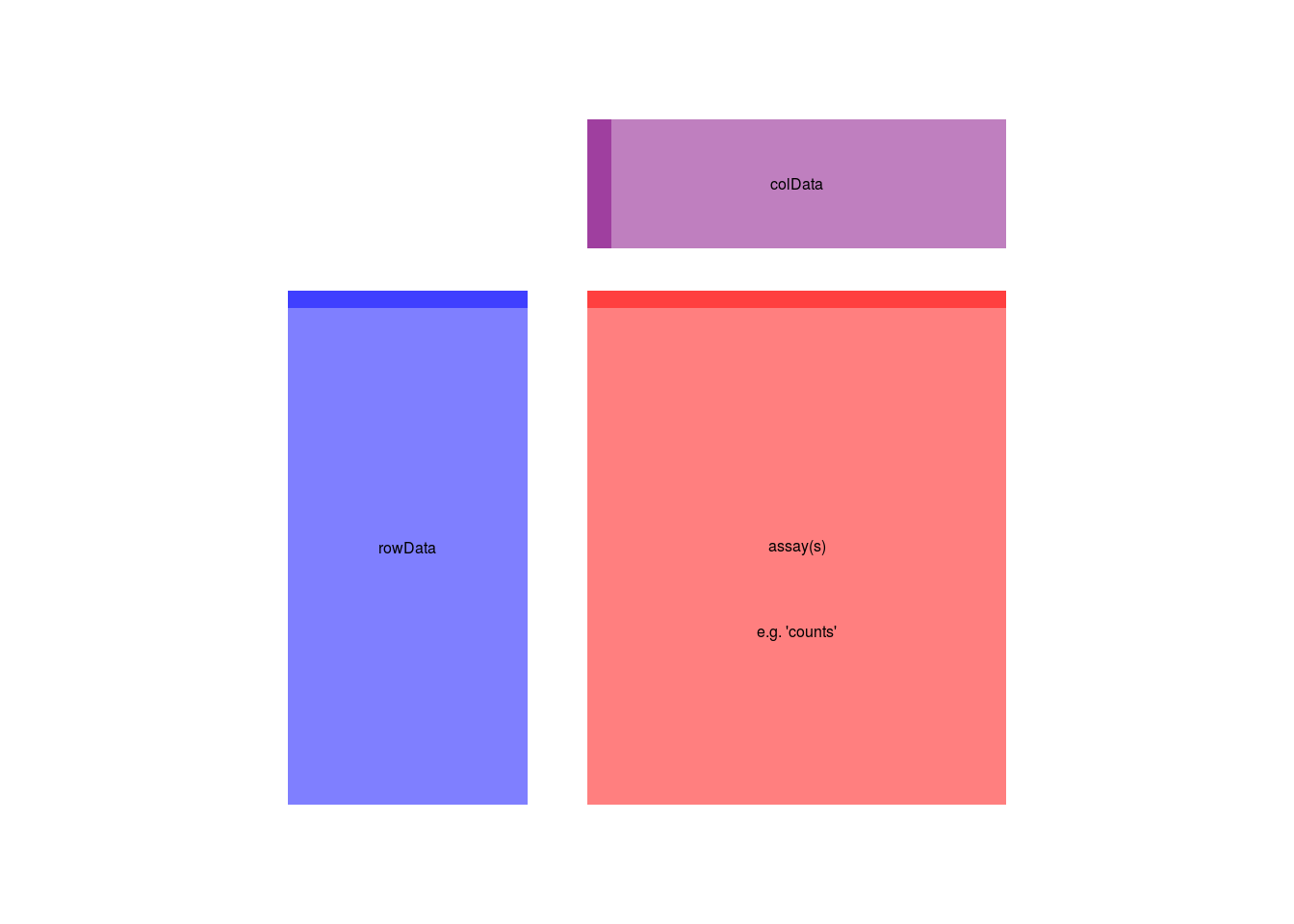Here we show the component parts of a SummarizedExperiment object, and also its subclasses, such as the DESeqDataSet which we downloaded.

The assay(s) (red block) contains the matrix (or matrices) of summarized values, the rowData (blue block) contains information about the genomic ranges, and the colData (purple block) contains information about the samples or experiments. The highlighted line in each block represents the first row (note that the first row of colData lines up with the first column of the assay.

We can investigate the content of the downloaded DESeqDataSet by looking at the counts in the assay slot, the phenotypic data about the samples in colData (sample information), and the data about the genes in the rowData (information on genomic features) slot.

DESeq2Table
## class: DESeqDataSet
## dim: 37991 8
## assays(1): counts
## rownames(37991): ENSMUSG00000000001 ENSMUSG00000000003 ... ENSMUSG00000093788
##   ENSMUSG00000093789
## rowData names(0):
## colnames(8): WT-SRR1042885 WT-SRR1042886 ... Del_8_17_homo-SRR1042891
##   Del_8_17_homo-SRR1042892
## colData names(3): condition sampleNO fastq
head(assay(DESeq2Table))
##                    WT-SRR1042885 WT-SRR1042886 WT-SRR1042887 WT-SRR1042888 Del_8_17_homo-SRR1042889
## ENSMUSG00000000001          2478          2010          4371          4222                     3115
## ENSMUSG00000000003             0             0             0             0                        0
## ENSMUSG00000000028           492           377           913           909                      647
## ENSMUSG00000000031         15493          9791         21816         21350                    16111
## ENSMUSG00000000037           108            87           127           140                      114
## ENSMUSG00000000049             1             0             2             1                        0
##                    Del_8_17_homo-SRR1042890 Del_8_17_homo-SRR1042891 Del_8_17_homo-SRR1042892
## ENSMUSG00000000001                     1346                     2599                     7895
## ENSMUSG00000000003                        0                        0                        0
## ENSMUSG00000000028                      265                      542                     1674
## ENSMUSG00000000031                     7307                    14793                    40392
## ENSMUSG00000000037                       43                       95                      301
## ENSMUSG00000000049                        0                        0                        4
colSums(assay(DESeq2Table))
##            WT-SRR1042885            WT-SRR1042886            WT-SRR1042887            WT-SRR1042888
##                  8080886                  6138993                 13637002                 13292699
## Del_8_17_homo-SRR1042889 Del_8_17_homo-SRR1042890 Del_8_17_homo-SRR1042891 Del_8_17_homo-SRR1042892
##                  9796176                  4272191                  8337066                 25035865
colData(DESeq2Table)
## DataFrame with 8 rows and 3 columns
##                              condition   sampleNO               fastq
##                               <factor>   <factor>            <factor>
## WT-SRR1042885                       WT SRR1042885 SRR1042885.fastq.gz
## WT-SRR1042886                       WT SRR1042886 SRR1042886.fastq.gz
## WT-SRR1042887                       WT SRR1042887 SRR1042887.fastq.gz
## WT-SRR1042888                       WT SRR1042888 SRR1042888.fastq.gz
## Del_8_17_homo-SRR1042889 Del_8_17_homo SRR1042889 SRR1042889.fastq.gz
## Del_8_17_homo-SRR1042890 Del_8_17_homo SRR1042890 SRR1042890.fastq.gz
## Del_8_17_homo-SRR1042891 Del_8_17_homo SRR1042891 SRR1042891.fastq.gz
## Del_8_17_homo-SRR1042892 Del_8_17_homo SRR1042892 SRR1042892.fastq.gz
rowData(DESeq2Table)
## DataFrame with 37991 rows and 0 columns

Note that the rowData slot is a GRangesList, which contains all the information about the exons for each gene, i.e., for each row of the count matrix. It also contains additional annotation for each gene, i.e. a gene description and a gene symbol.

mcols(rowData(DESeq2Table))
## DataFrame with 0 rows and 2 columns

The colData slot contains all the sample metadata.

colData(DESeq2Table)
## DataFrame with 8 rows and 3 columns
##                              condition   sampleNO               fastq
##                               <factor>   <factor>            <factor>
## WT-SRR1042885                       WT SRR1042885 SRR1042885.fastq.gz
## WT-SRR1042886                       WT SRR1042886 SRR1042886.fastq.gz
## WT-SRR1042887                       WT SRR1042887 SRR1042887.fastq.gz
## WT-SRR1042888                       WT SRR1042888 SRR1042888.fastq.gz
## Del_8_17_homo-SRR1042889 Del_8_17_homo SRR1042889 SRR1042889.fastq.gz
## Del_8_17_homo-SRR1042890 Del_8_17_homo SRR1042890 SRR1042890.fastq.gz
## Del_8_17_homo-SRR1042891 Del_8_17_homo SRR1042891 SRR1042891.fastq.gz
## Del_8_17_homo-SRR1042892 Del_8_17_homo SRR1042892 SRR1042892.fastq.gz

At this point, we have counted the reads which overlap the genes in the gene model we specified. Note that the class DataFrame is a Bioconductor variant of the standard data.frame class in R. which has among other things a more sensible print funtion, only showing the head and the tail of the table.

Before we proceed, now correct an annotation error: the samples SRR1042891 and SRR1042886 have the opposite genotype

con <- as.character(colData(DESeq2Table)$condition) con <- "Del_8_17_homo" con <- "WT" colData(DESeq2Table)$condition <- factor(con)

We can now move on to the actual differential expression analysis steps after specifying an appropriate design.

# 5 Experimental design and the design formula

The DESeqDataSet has an associated design formula. The design is specified at the beginning of the analysis, as it will inform many of the DESeq2 functions how to treat the samples in the analysis (one exception is the size factor estimation, i.e., the adjustment for differing library sizes, which does not depend on the design formula). The design formula tells which variables in the column metadata table (colData) specify the experimental design and how these factors should be used in the analysis.

The simplest design formula for differential expression would be ~ condition, where condition is a column in colData(DESeq2Table) which specifies which of two (or more groups) the samples belong to. This is also what we will be using for the data at hand.

You can use R’s formula notation to express any experimental design that can be described within an ANOVA–like framework. Note that DESeq2 uses the same formula notation as, for instance, the lm function of base R. If the question of interest is whether a fold change due to treatment is different across groups, interaction terms can be included using models such as ~ group + treatment + group:treatment. More complex designs such as these are covered in the DESeq2 vignette.

# 6 Quality control and Normalization of the count data

Having created a count table and fed it into a DESeq2 object, the next step in the workflow is the quality control of the data. Let’s check how many genes we capture by counting the number of genes that have non–zero counts in all samples.

GeneCounts <- counts(DESeq2Table)
idx.nz <- apply(GeneCounts, 1, function(x) { all(x > 0)})
sum(idx.nz)
##  16149
### random sample from the count matrix
nz.counts <- subset(GeneCounts, idx.nz)
sam <- sample(dim(nz.counts), 5)
nz.counts[sam, ]
##                    WT-SRR1042885 WT-SRR1042886 WT-SRR1042887 WT-SRR1042888 Del_8_17_homo-SRR1042889
## ENSMUSG00000037363            38            15            58            52                       47
## ENSMUSG00000029060           333           255           498           554                      381
## ENSMUSG00000078247            58            57            71            96                       73
## ENSMUSG00000045095           644           531          1105          1117                      800
## ENSMUSG00000071506             2             9            11            12                       11
##                    Del_8_17_homo-SRR1042890 Del_8_17_homo-SRR1042891 Del_8_17_homo-SRR1042892
## ENSMUSG00000037363                       23                       36                      100
## ENSMUSG00000029060                      175                      326                      951
## ENSMUSG00000078247                       37                       57                      190
## ENSMUSG00000045095                      336                      682                     2124
## ENSMUSG00000071506                        4                        8                       19

In a typical RNA-Seq experiment, there will be at least several thousand genes that are expressed in all samples. If the number of non–zero genes is very low, there is usually something wrong with at least some of the samples (e.g. the sample preparation was not done properly, or there was some problem with the sequencing).

# 7 Normalization

As different libraries will be sequenced to different depths, offsets are built in the statistical model of DESeq2 to ensure that parameters are comparable.

The term normalization is often used for that, but it should be noted that the raw read counts are not actually altered. DESeq2 defines a virtual reference sample by taking the median of each gene’s values across samples and then computes size factors as the median of ratios of each sample to the reference sample.

Generally, the ratios of the size factors should roughly match the ratios of the library sizes. Dividing each column of the count table by the corresponding size factor yields normalized count values, which can be scaled to give a counts per million interpretation.

Thus, if all size factors are roughly equal to one, the libraries have been sequenced equally deeply.

Count data sets typically show a (left–facing) trombone shape in average vs mean–difference plots (MA–plots), reflecting the higher variability of log ratios at lower counts. In addition, points will typically be centered around a log ratio of 0 if the normalization factors are calculated appropriately, although this is just a general guide.

In the code below we produce density estimates of the sample counts and pairwise mean–difference plots after the computation of the size factors. The MA–plots are saved to a file in order to avoid cluttering the document.

We first relevel the condition factor to make sure that we have the wild type samples as a base level. This will allow us to obtain the fold changes always as WT–deletion. We also rename the deletion level to “Del”.

colData(DESeq2Table)$condition <- factor(colData(DESeq2Table)$condition, levels = c("WT", "Del"))
colData(DESeq2Table)$condition <- factor(ifelse(is.na(colData(DESeq2Table)$condition),  "Del", "WT"), levels = c("WT", "Del"))

We now estimate estimate the size factors an print them

DESeq2Table <- estimateSizeFactors(DESeq2Table)
sizeFactors(DESeq2Table)
##            WT-SRR1042885            WT-SRR1042886            WT-SRR1042887            WT-SRR1042888
##                   0.8362                   0.6322                   1.3975                   1.3709
## Del_8_17_homo-SRR1042889 Del_8_17_homo-SRR1042890 Del_8_17_homo-SRR1042891 Del_8_17_homo-SRR1042892
##                   1.0086                   0.4473                   0.8602                   2.6086

To assess whether the normalization has worked, we plot the densities of counts for the different samples. Since most of the genes are (heavily) affected by the experimental conditions, a succesful normalization will lead to overlapping densities.

multidensity( counts(DESeq2Table, normalized = T)[idx.nz ,],
xlab="mean counts", xlim=c(0, 1000))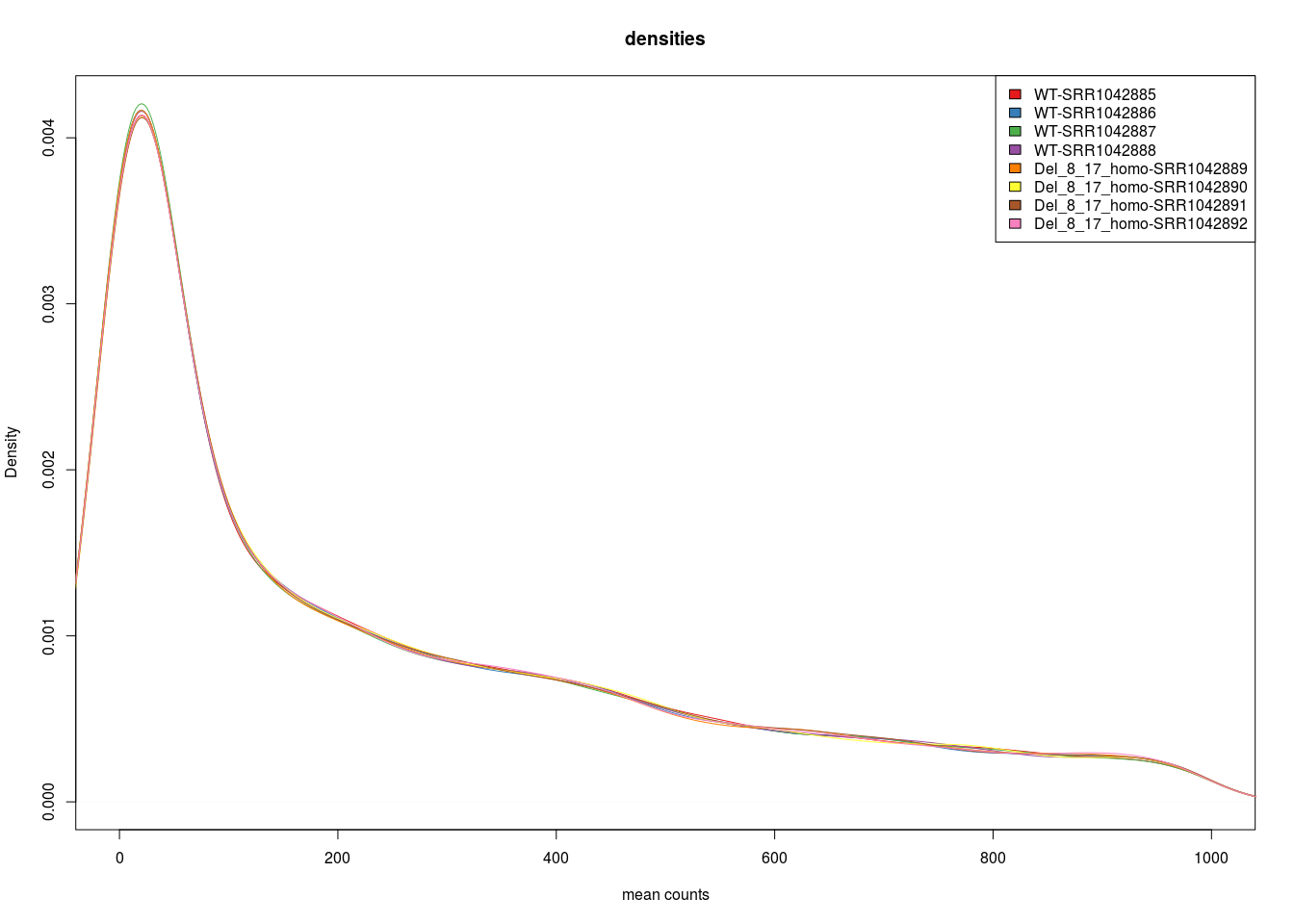This is indeed the case here. The same holds true for the empircal cummulative distribution functions (ECDFs) which can be thought of as integrals of the densities and give the probability of observing a certain number of counts equal to $$x$$ or less given the data.

In an ECDF plot, the estimated probility is plotted on the y–axis and the count values on the x–axis. I.e. you can read of the median and other quantiles from this plot.

As already mentioned, if the normalization has worked, the ECDFs of the different samples should be overlapping.

 multiecdf( counts(DESeq2Table, normalized = T)[idx.nz ,],
xlab="mean counts", xlim=c(0, 1000))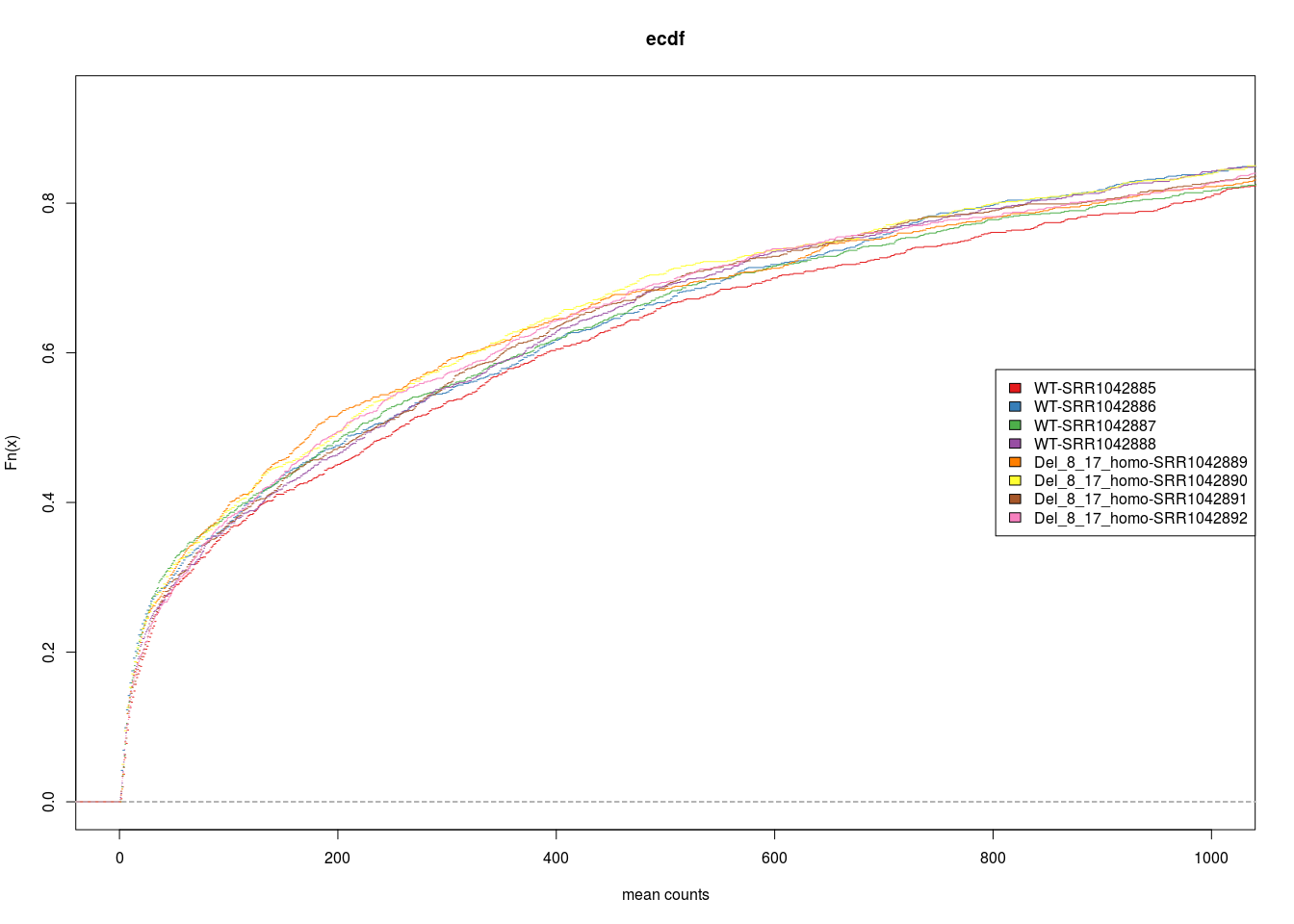To further assess systematic differences between the samples, we can also plot pairwise mean–average plots: We plot the average of the log–transformed counts vs the fold change per gene for each of the sample pairs.

The function combn helps us to automatically generate all sample pairs and the function MDPlot from the EDASeq package then generates the the pairwise MA plots.

pdf("pairwiseMAs.pdf")
MA.idx = t(combn(1:8, 2))
for( i in  seq_along( MA.idx[,1])){
MDPlot(counts(DESeq2Table, normalized = T)[idx.nz ,],
c(MA.idx[i,1],MA.idx[i,2]),
main = paste( colnames(DESeq2Table)[MA.idx[i,1]], " vs ",
colnames(DESeq2Table)[MA.idx[i,2]] ), ylim = c(-3,3))
}
dev.off()
## png
##   2

The plots look good, if there is no systematic shift between the samples, the log–fold–change should scatter around zero, which is the case here.

# 8 PCA and sample heatmaps

A useful first step in an RNA–Seq analysis is often to assess overall similarity between samples: Which samples are similar to each other, which are different? Does this fit to the expectation from the experiment’s design? We use the function to calculate the Euclidean distance between samples. To avoid that the distance measure is dominated by a few highly variable genes, and have a roughly equal contribution from all genes, we use it on the regularized log–transformed data.

The aim of the regularized log–transform is to stabilize the variance of the data and to make its distribution roughly symmetric since many common statistical methods for exploratory analysis of multidimensional data, especially methods for clustering and ordination (e.g., principal–component analysis and the like), work best for (at least approximately) homoskedastic data; this means that the variance of an observable quantity (i.e., here, the expression strength of a gene) does not depend on the mean.

In RNA–Seq data, however, variance grows with the mean. For example, if one performs PCA directly on a matrix of normalized read counts, the result typically depends only on the few most strongly expressed genes because they show the largest absolute differences between samples.

A simple and often used strategy to avoid this is to take the logarithm of the normalized count values plus a small pseudocount; however, now the genes with low counts tend to dominate the results because, due to the strong Poisson noise inherent to small count values, they show the strongest relative differences between samples. Note that this effect can be diminished by adding a relatively high number of pseudocounts, e.g. 32, since this will also substantially reduce the variance of the fold changes.

As a solution, DESeq2 offers the regularized–logarithm transformation, or rlog for short. For genes with high counts, the rlog transformation differs not much from an ordinary log2 transformation.

For genes with lower counts, however, the values are shrunken towards the genes’ averages across all samples. Using an empirical Bayesian prior in the form of a ridge penalty, this is done such that the rlog–transformed data are approximately homoskedastic. Note that the rlog transformation is provided for applications other than differential testing. For differential testing it is always recommended to apply the DESeq function to raw counts.

Note the use of the function to transpose the data matrix. We need this because dist calculates distances between data rows and our samples constitute the columns. We visualize the distances in a heatmap, using the function heatmap.2 from the r CRANpkg("gplots") package.

Note that we have changed the row names of the distance matrix to contain the genotype and the column to contain the sample ID, so that we have all this information in view when looking at the heatmap.

rld <- rlogTransformation(DESeq2Table, blind=TRUE)

distsRL <- dist(t(assay(rld)))
mat <- as.matrix(distsRL)
rownames(mat) <-  colData(rld)$condition colnames(mat) <- colData(rld)$sampleNO

hmcol <- colorRampPalette(brewer.pal(9, "Blues"))(255)
heatmap.2(mat, trace="none", col = rev(hmcol), margin=c(13, 13))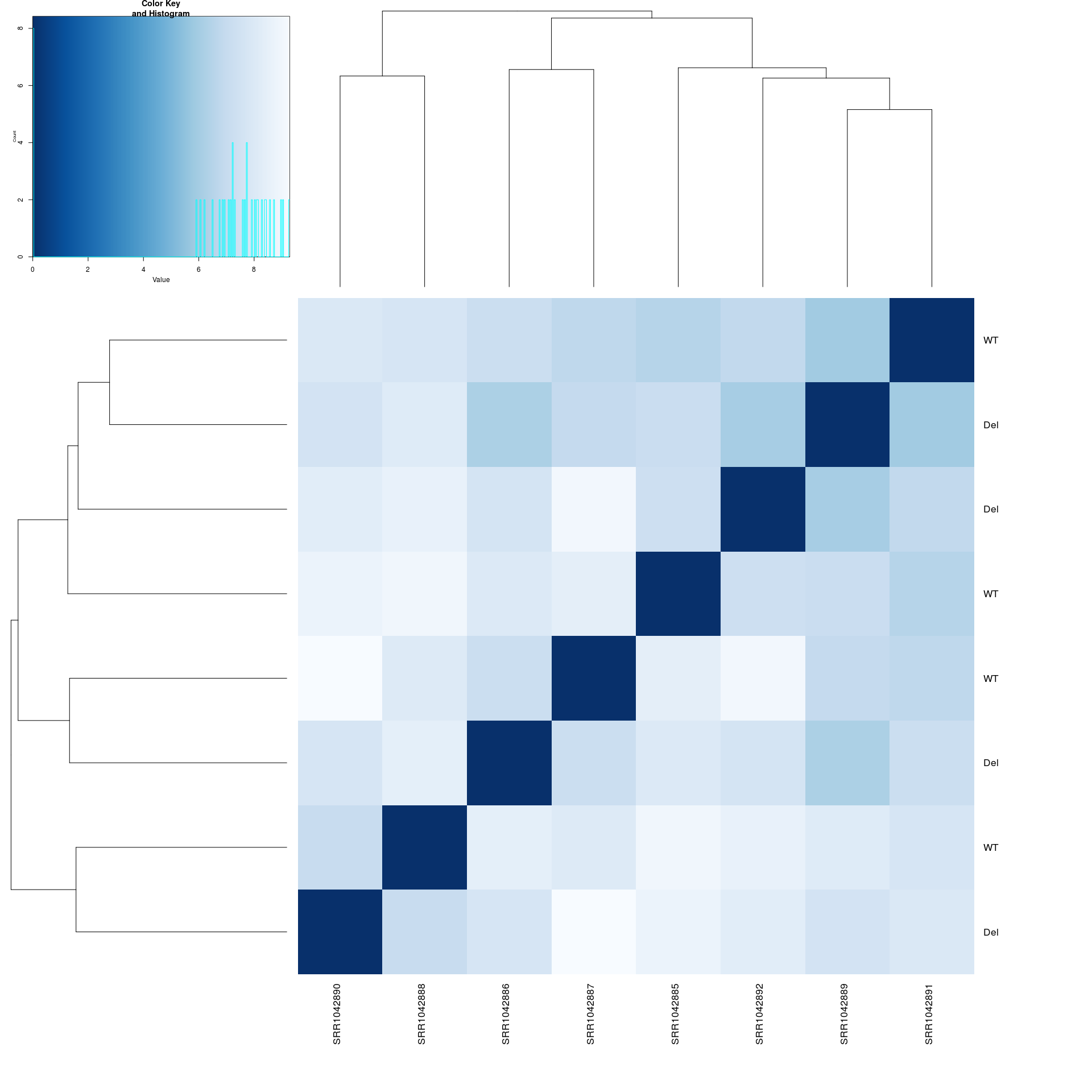Another way to visualize sample–to–sample distances is a principal–components analysis (PCA). In this ordination method, the data points (i.e., here, the samples) are projected onto the 2D plane such that they spread out optimally.

ntop = 500

Pvars <- rowVars(assay(rld))
select <- order(Pvars, decreasing = TRUE)[seq_len(min(ntop,
length(Pvars)))]

PCA <- prcomp(t(assay(rld)[select, ]), scale = F)
percentVar <- round(100*PCA$sdev^2/sum(PCA$sdev^2),1)

Since PCA can be slightly problematic with high dimensional data, we first use select only the 500 genes showing the highest variance.

We then use the function prcomp to compute the principal components. The resulting principal component scores, i.e. the coordinates of the samples in the space of the principal components can then be plotted.

For this we use the function qplot from ggplot2. ggplot2 is a comprehensive plotting package for R, a very good tutorial is here. The function qplot is supposed to mimic the standard plotting function plot as closely as possible, but additional changes can be made, e.g. lattice–type graphics (splitting the plot by a factor of interest) can easily be generated.

Addition elements are changed by using + and the corresponding commands. In our PCA plot, we change the plotting colors used and the axis labels.

dataGG = data.frame(PC1 = PCA$x[,1], PC2 = PCA$x[,2],
PC3 = PCA$x[,3], PC4 = PCA$x[,4],
sampleNO = colData(rld)$sampleNO, condition = colData(rld)$condition)

(qplot(PC1, PC2, data = dataGG, color =  condition,
main = "PC1 vs PC2, top variable genes", size = I(6))
+ labs(x = paste0("PC1, VarExp:", round(percentVar,4)),
y = paste0("PC2, VarExp:", round(percentVar,4)))
+ scale_colour_brewer(type="qual", palette=2)
)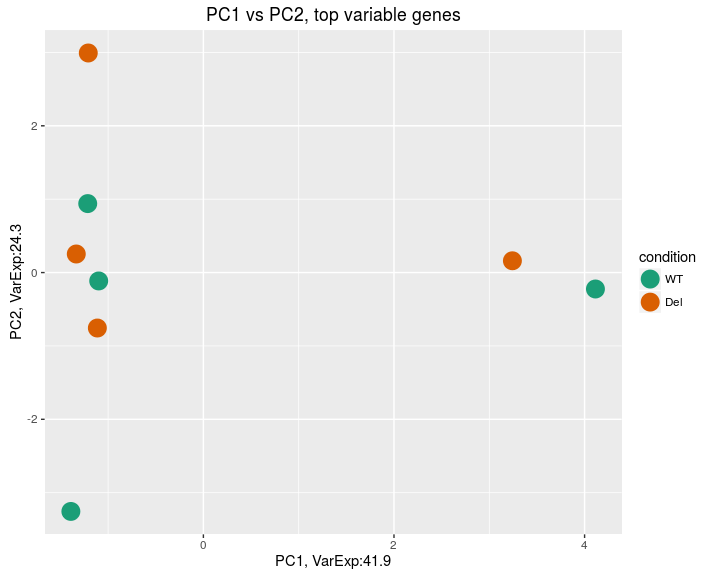We can clearly identify to outliers in the PCA plot, one in each experimental groups. These two outliers basically provide the separation according to the first principal component. Thus, they outliers will most likely increase the overall variability and thus diminish statistical power later when testing for differential expression. Therefore we remove them. We can get them by selecting only those samples that have a value greater than zero for the first principal component score. This can conveniently be done using the function subset and specifying the logical vector dataGG$PC1 > 0 . subset will then return the sample names a positions where dataGG$PC1 > 0 evaluates to TRUE.

outliers <- as.character(subset(colnames(DESeq2Table), dataGG$PC1 > 0)) outliers ##  "WT-SRR1042888" "Del_8_17_homo-SRR1042890" DESeq2Table <- DESeq2Table[, !(colnames(DESeq2Table) %in% outliers)]  We now produce the cluster heatmap and the PCA plot without the outliers. rld <- rlogTransformation(DESeq2Table, blind=TRUE) distsRL <- dist(t(assay(rld))) mat <- as.matrix(distsRL) rownames(mat) <- colData(rld)$condition
colnames(mat) <-  colData(rld)$sampleNO hmcol <- colorRampPalette(brewer.pal(9, "OrRd"))(255) heatmap.2(mat, trace="none", col = rev(hmcol), margin=c(13, 13))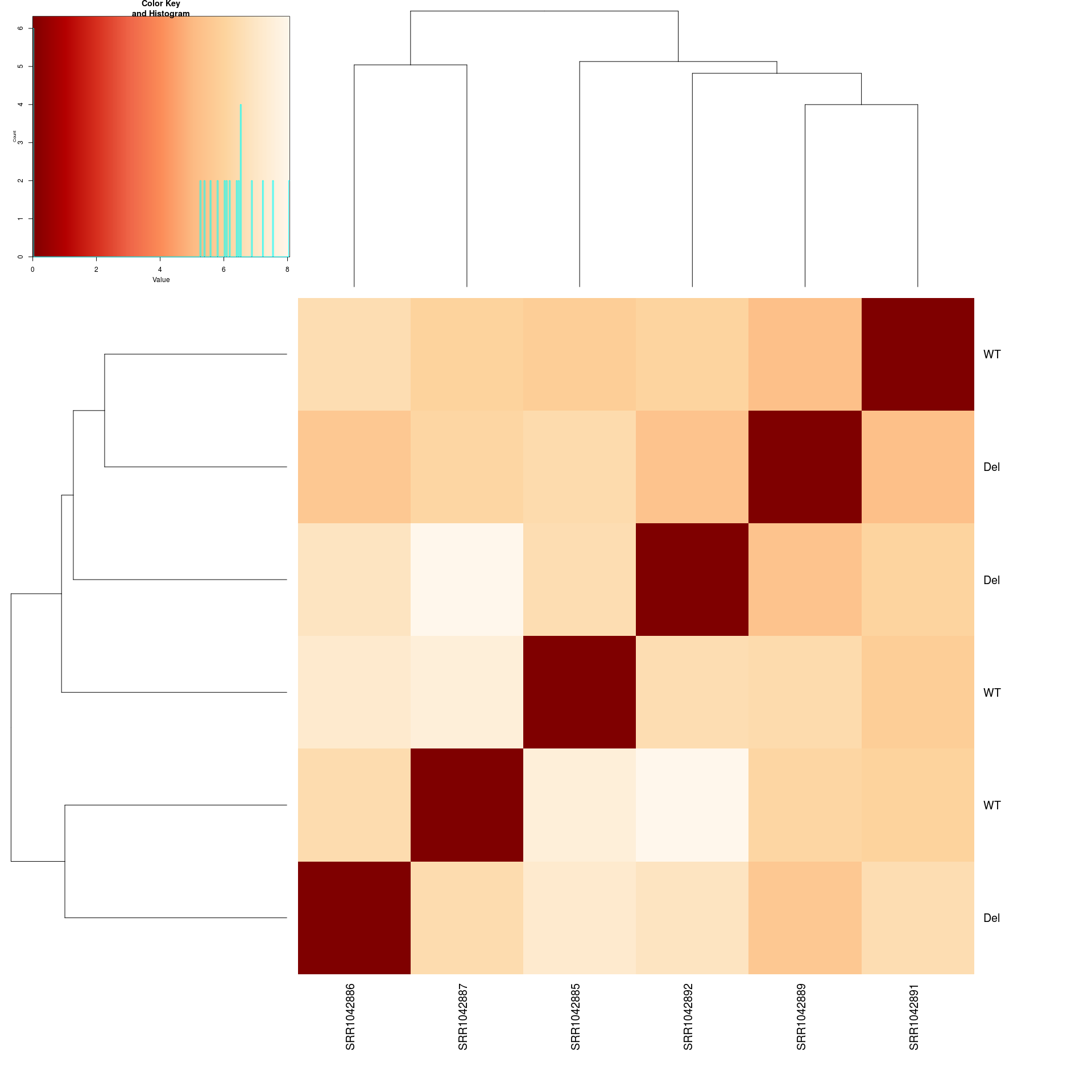Pvars <- rowVars(assay(rld)) select <- order(Pvars, decreasing = TRUE)[seq_len(min(ntop, length(Pvars)))] PCA <- prcomp(t(assay(rld)[select, ]), scale = F) percentVar <- round(100*PCA$sdev^2/sum(PCA$sdev^2),1) dataGG = data.frame(PC1 = PCA$x[,1], PC2 = PCA$x[,2], PC3 = PCA$x[,3], PC4 = PCA$x[,4], sampleNO = colData(rld)$sampleNO,
condition = colData(rld)$condition) (qplot(PC1, PC2, data = dataGG, color = condition, main = "PC1 vs PC2, top variable genes, no outliers", size = I(6)) + labs(x = paste0("PC1, VarExp:", round(percentVar,4)), y = paste0("PC2, VarExp:", round(percentVar,4))) + scale_colour_brewer(type="qual", palette=4) )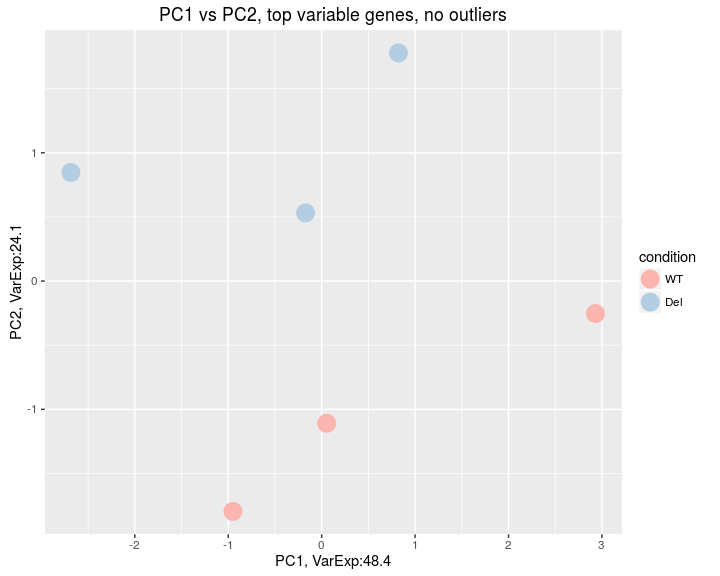The separation between the two groups is not extremely clear, they do not cluster together in the heatmap and they also are only separated by the second principal component. This will most likely result in only a modest number of differentially expressed genes and is in line with the small number of differentially expressed genes (~ 100) the authors report in the original publication. # 9 Differential expression analysis It is known that a standard Poisson model can only account for the technical noise in RNA–Seq data. In the Poisson model the variance is equal to the mean, while in real RNA–Seq data the variance is greater than the mean, a phenomenon often encountered in count data in general and referred to as “overdispersion”. A popular way to model this is to use a Negative–Binomial-distribution (NB), which includes an additional parameter dispersion parameter $$\alpha$$ such that $$E(NB) = \mu$$ and $\text{Var[NB(\mu, \alpha)]} = \mu + \alpha \cdot \mu^2$ Hence, the variance is greater than the mean. DESeq2 also uses the NB model. Hence, the first step in the analysis of differential expression, is to obtain an estimate of the dispersion parameter for each gene. The typical shape of the dispersion fit is an exponentially decaying curve. The asymptotic dispersion for highly expressed genes can be seen as a measurement of biological variability in the sense of a squared coefficient of variation: a dispersion value of 0.01 means that the gene’s expression tends to differ by typically $$\sqrt{0.01}$$ = 10% between samples of the same treatment group. For weak genes, the Poisson noise is an additional source of noise, which is added to the dispersion. The function visualizes DESeq2’s dispersion estimates: DESeq2Table <- estimateDispersions(DESeq2Table) plotDispEsts(DESeq2Table)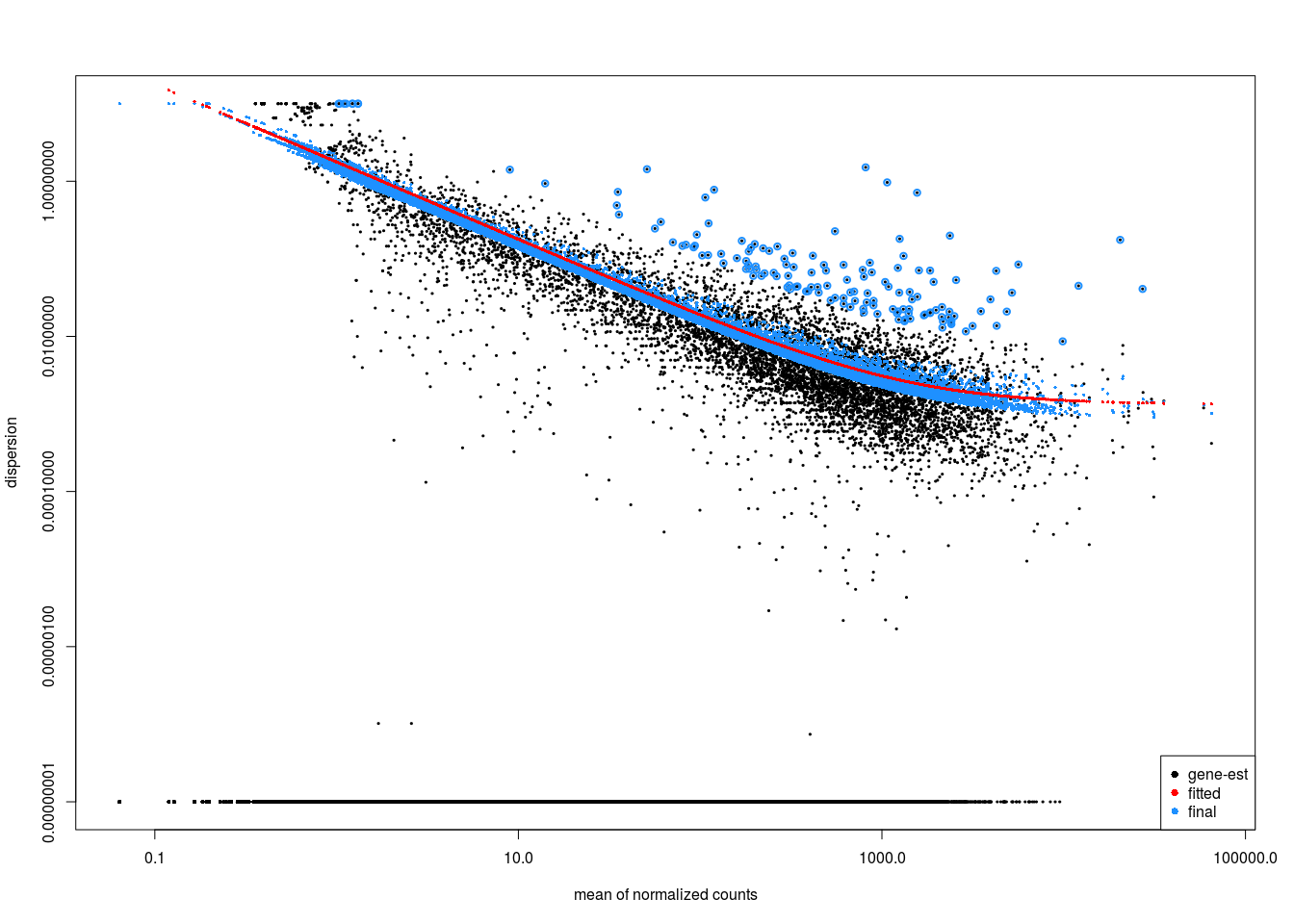The black points are the dispersion estimates for each gene as obtained by considering the information from each gene separately. Unless one has many samples, these values fluctuate strongly around their true values. Therefore, the red trend line is fitted, which shows the dispersions’ dependence on the mean, and then shrink each gene’s estimate towards the red line to obtain the final estimates (blue points) that are then used in the hypothesis test. The blue circles above the main “cloud” of points are genes which have high gene–wise dispersion estimates which are labelled as dispersion outliers. These estimates are therefore not shrunk toward the fitted trend line. The warnings just indicate that the dispersion estimation failed for some genes. # 10 Statistical testing of Differential expression We can perform the statistical testing for differential expression and extract its results. Calling performs the test for differential expression, while the call to the function extracts the results of the test and returns adjusted $$p$$–values according to the Benjamini–Hochberg rule to control the FDR. The test–performed is Wald test, which is a test for coefficients in a regression model. It is based on a z–score, i.e. a $$N(0,1)$$ distributed (under $$H_0$$) test statistic. DESeq2Table <- nbinomWaldTest(DESeq2Table) DESeq2Res <- results(DESeq2Table, pAdjustMethod = "BH") table(DESeq2Res$padj < 0.1)
##
## FALSE  TRUE
## 17525   147

We identify 147 differentially expressed genes.

## 10.1 Independent filtering

In addition to this, DESeq2 performs automated independent filtering: For weakly expressed genes, we have no chance of seeing differential expression, because the low read counts suffer from so high Poisson noise that any biological effect is drowned in the uncertainties from the read counting.

At first sight, there may seem to be little benefit in filtering out these genes. After all, the test found them to be non–significant anyway. However, these genes have an influence on the multiple testing procedure, whose performance commonly improves if such genes are removed. By removing the weakly–expressed genes from the input to the BH–FDR procedure, we can find more genes to be significant among those which we keep, and so improve the power of our test. This approach is known as independent filtering.

The DESeq2 software automatically performs independent filtering which maximizes the number of genes which will have a BH–adjusted $$p$$–value less than a critical value (by default, alpha is set to 0.1). This automatic independent filtering is performed by, and can be controlled by, the results function. We can observe how the number of rejections changes for various cutoffs based on mean normalized count. The following optimal threshold and table of possible values is stored as an attribute of the results object.

plot(metadata(DESeq2Res)$filterNumRej, type="b", xlab="quantiles of 'baseMean'", ylab="number of rejections")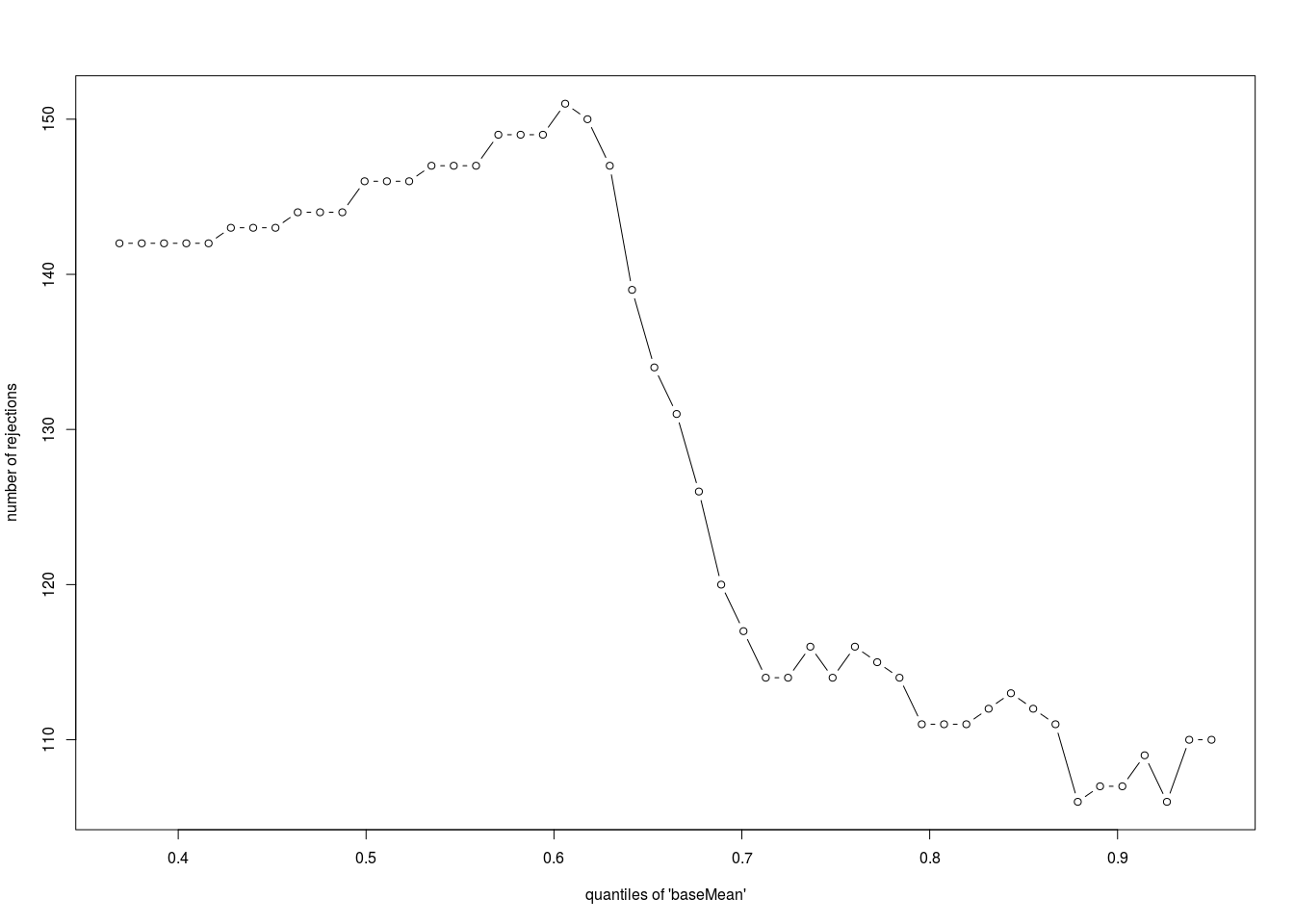The term independent highlights an important caveat. Such filtering is permissible only if the filter criterion is independent of the actual test statistic under the null–hypothesis. Otherwise, the filtering would invalidate the test and consequently the assumptions of the BH procedure. This is why we filtered on the average over all samples: this filter is blind to the assignment of samples to the deletion and control group and hence independent under the null hypothesis of equal expression. ## 10.2 Inspection and correction of $$p$$–values The null–$$p$$–values follow a uniform distribution on the unit interval [0,1] if they are computed using a continuous null distribution. Significant $$p$$–values thus become visible as an enrichment of $$p$$–values near zero in the histogram. Thus, $$p$$–value histogram of “correctly” computed $$p$$–values will have a rectangular shape with a peak at 0. A histogram of $$p$$–values should always be plotted in order to check whether they have been computed correctly. We also do this here: hist(DESeq2Res$pvalue, col = "lavender", main = "WT vs Deletion", xlab = "p-values")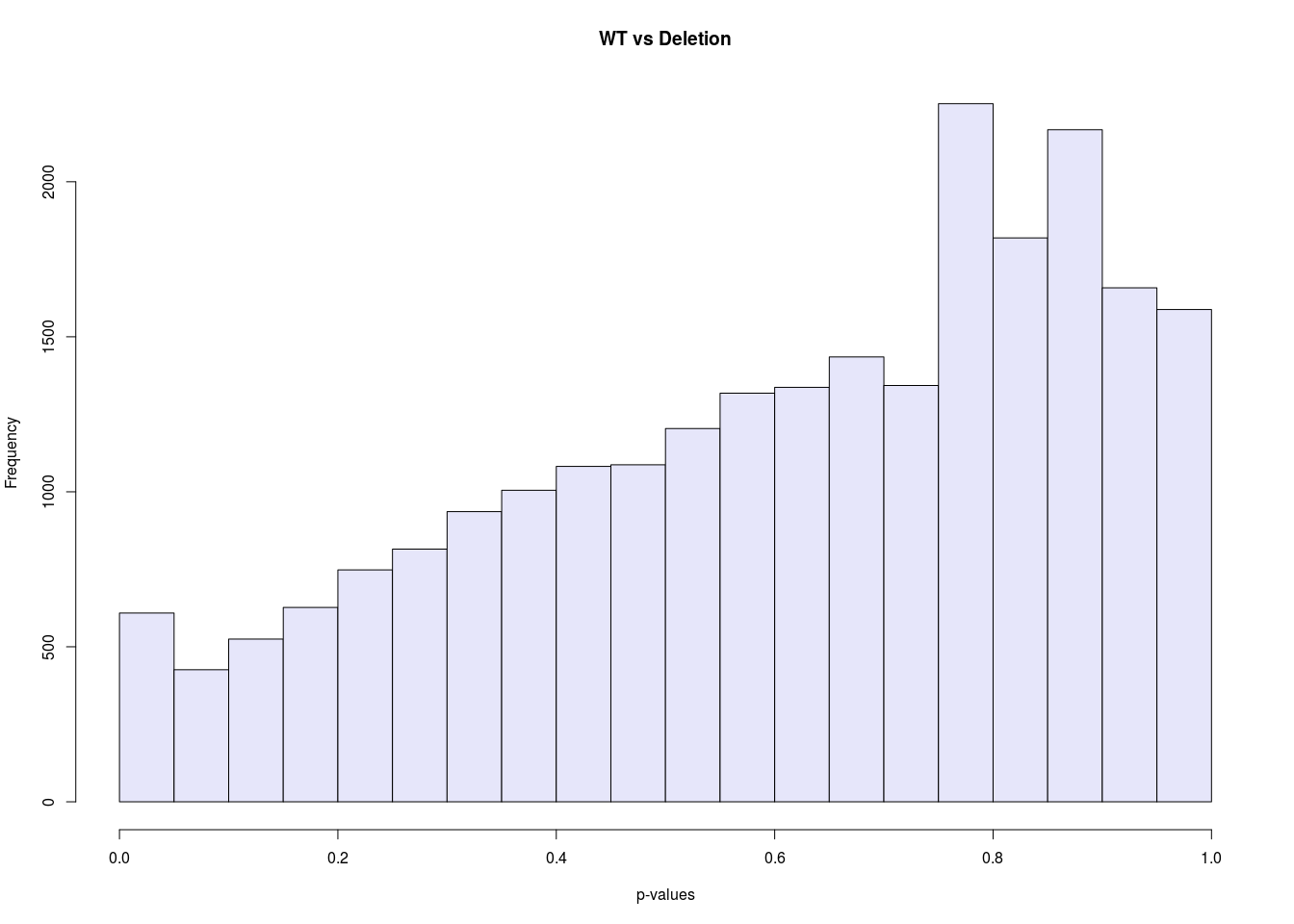We can see that this is clearly not the case for the $$p$$–values returned by DESeq2 in this case.

Very often, if the assumed variance of the null distribution is too high, we see hill–shaped $$p$$–value histogram. If the variance is too low, we get a U–shaped histogram, with peaks at both ends.

Here we have a hill–shape, indicating an overestimation of the variance in the null distribution. Thus, the $$N(0,1)$$ null distribution of the Wald test is not appropriate here.

The dispersion estimation is not condition specific and estimates only a single dispersion estimate per gene. This is sensible, since the number of replicates is usually low.

However, if we have e.g. batches or “outlying” samples that are consistently a bit different from others within a group, the dispersion within the experimental group can be different and a single dispersion parameter not be appropriate.

For an example of the estimation of multiple dispersions, see the analysis performed in: Reyes et. al. - Drift and conservation of differential exon usage across tissues in primate species, 2013

Fortunately, there is software available to estimate the variance of the null–model from the test statistics. This is commonly referred to as “empirical null modelling”.

Here we use the fdrtool for this using the Wald statistic as input. This packages returns the estimated null variance, as well as estimates of various other FDR–related quantities and the $$p$$–values computed using the estimated null model parameters.

We first remove genes filtered out by independent filtering and the dispersion outliers, they have NA adj. pvals and NA $$p$$–values respectively.

DESeq2Res <- DESeq2Res[ !is.na(DESeq2Res$padj), ] DESeq2Res <- DESeq2Res[ !is.na(DESeq2Res$pvalue), ]

We now remove the original adjusted $$p$$–values, since we will add the corrected ones later on.

DESeq2Res <- DESeq2Res[, -which(names(DESeq2Res) == "padj")]

We can now use z–scores returned by DESeq2as input to fdrtool to re–estimate the $$p$$–values

FDR.DESeq2Res <- fdrtool(DESeq2Res$stat, statistic= "normal", plot = T) ## Step 1... determine cutoff point ## Step 2... estimate parameters of null distribution and eta0 ## Step 3... compute p-values and estimate empirical PDF/CDF ## Step 4... compute q-values and local fdr ## Step 5... prepare for plotting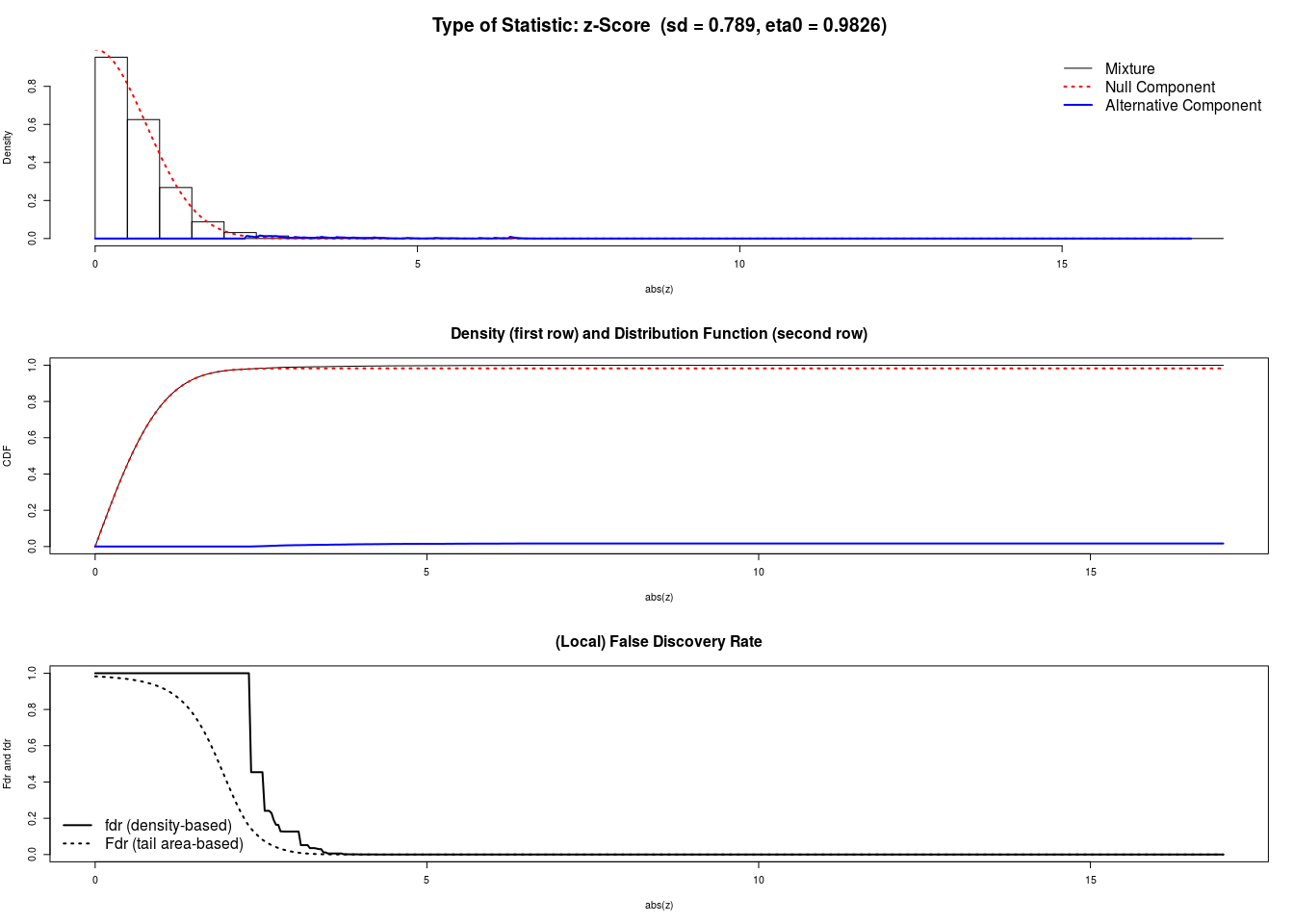FDR.DESeq2Res$param[1, "sd"]
##     sd
## 0.7888

Interestingly the estimated null model variance is 0.7888, which is lower than $$1$$, the theoretical one.

We now add the new BH–adjusted $$p$$–values add values to the results data frame.

DESeq2Res[,"padj"]  <- p.adjust(FDR.DESeq2Res$pval, method = "BH") We can now plot the histogram of the “correct” $$p$$–values. hist(FDR.DESeq2Res$pval, col = "royalblue4",
main = "WT vs Deletion, correct null model", xlab = "CORRECTED p-values")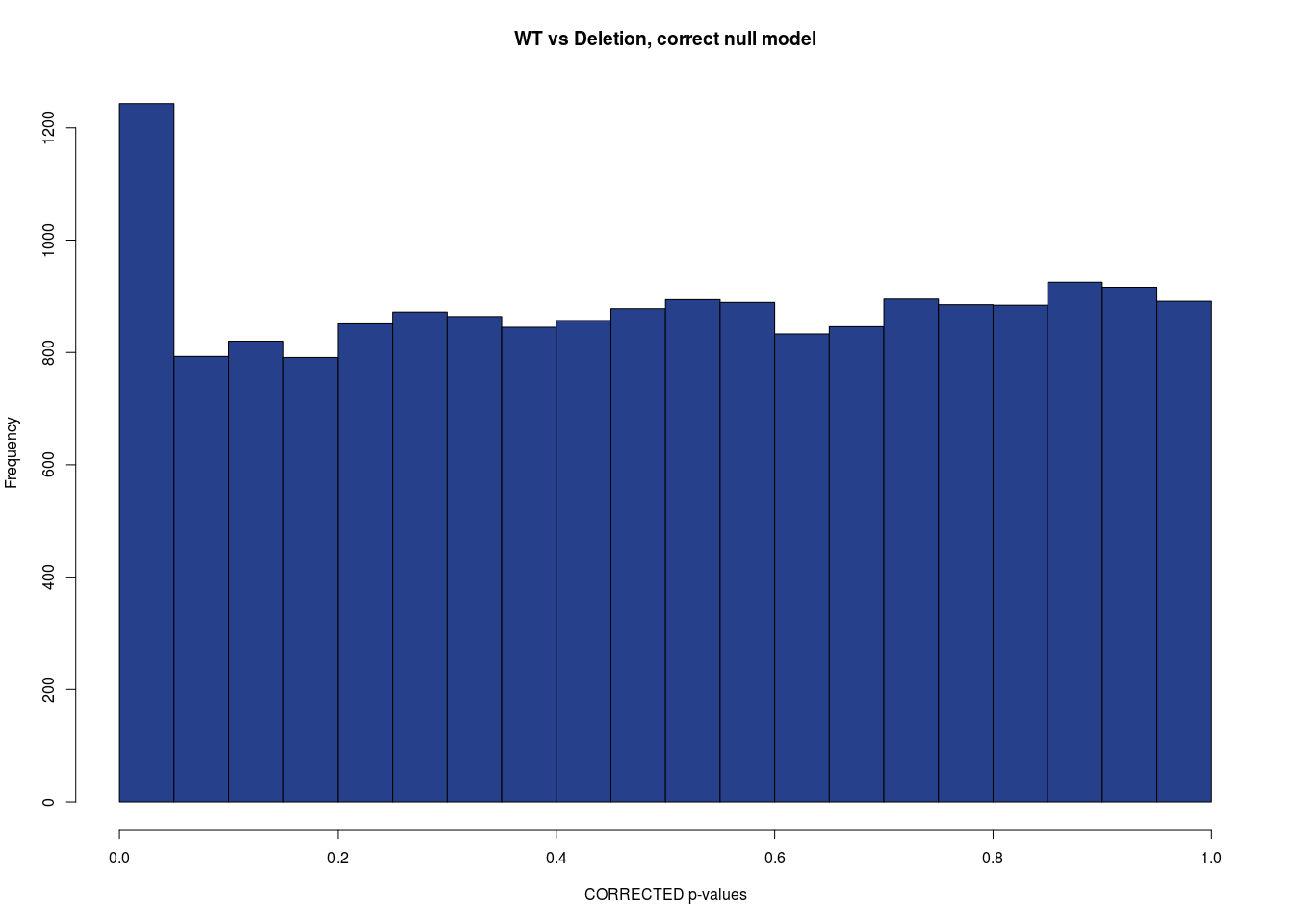As we can see, the null model is now correct and the histogram has the expected shape.

## 10.3 Extracting differentially expressed genes

We can now extract the number of differentially expressed genes and produce an MA plot of the two–group comparison as shown in figure 4a of the original paper. (“M” for minus, because a log ratio is equal to log minus log, and “A” for average).

Similar to its usage in the initial quality control, a MA plot provides a useful overview for an experiment with a two-group comparison:

The plot represents each gene with a dot. The $$x$$–axis is the average expression over all samples, the y axis the log2 fold change between treatment and control. Genes with an FDR value below a threshold (here 0.1) are shown in red. This plot demonstrates that only genes with a large average normalized count contain more information to yield a significant call.

Also note DESeq2’s shrinkage estimation of log fold changes (LFCs): When count values are too low to allow an accurate estimate of the LFC, the value is “shrunken” towards zero to avoid that these values, which otherwise would frequently be unrealistically large, dominate the top–ranked log fold changes. Thus, in contrast to the “trumpet shaped” of the MA plot in the original paper (they used the orginal DESeq, we obtain a “cigar-shaped” MA plot.

table(DESeq2Res[,"padj"] < 0.1)
##
## FALSE  TRUE
## 17369   303
plotMA(DESeq2Res)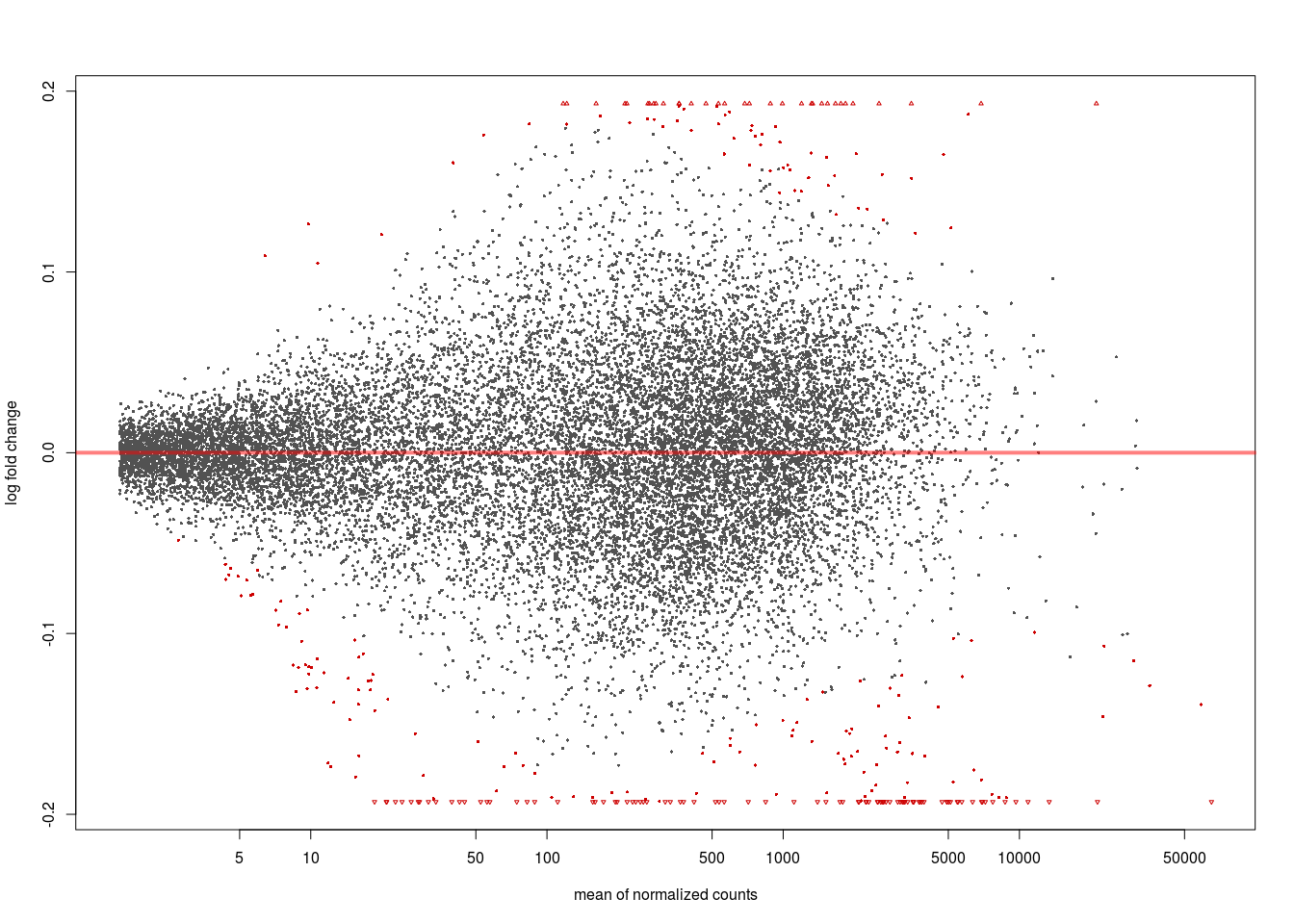We now identify 303 differentially expressed genes at an FDR of $$0.1$$.

## 10.4 Check overlap with the paper results

We can now check the overlap with the original results of the paper. In the original publication 100 genes with an FDR value of less than $$0.05$$ were identified. The excel table “ng.2971–S3.xls” containing their FC and $$p$$–values is available as supplementary table 4 of the original publication. We convert the table into a .csv file and import it.

paperRes <- read.csv("ng.2971-S3.csv", skip = 1, header =T)
names(paperRes)
##   "Gene_ID"        "baseMean"       "baseMean.WT"    "baseMean.DEL"   "fold.change"
##   "log2FoldChange" "pval"           "padj"           "GROUP"          "Gene.name"
##  "chr"            "start.mm10."    "end.mm10."      "strand"         "Gene.name.1"
##  "KEGG"           "GO"

We now extract our significant genes and their annotation

sigGenes <- rownames(subset(DESeq2Res, padj < 0.1))

anno <- AnnotationDbi::select(org.Mm.eg.db,
keys=rownames(DESeq2Res),
columns=c("SYMBOL","SYMBOL", "GENENAME"),
keytype="ENSEMBL")

anSig <- as.data.frame(subset(anno, ENSEMBL %in% sigGenes))

sample_n(anSig, 5)
##                  ENSEMBL SYMBOL                             GENENAME
## 11862 ENSMUSG00000047935 Gm5607                  predicted gene 5607
## 7750  ENSMUSG00000032399   Rpl4                 ribosomal protein L4
## 13496 ENSMUSG00000058799 Nap1l1 nucleosome assembly protein 1-like 1
## 14631 ENSMUSG00000069516   Lyz2                           lysozyme 2
## 11735 ENSMUSG00000047215   Rpl9                 ribosomal protein L9

We now extract the genes identified in the original paper that we call as differentially expressed.

overlap <- subset(paperRes, Gene.name  %in% anSig$SYMBOL ) dim(overlap) ##  231 17 Now it can be asked how many genes that we identify with FDR < 0.1 also had FDR < 0.1 in the original paper? and get the total number of genes in the original paper with FDR < 0.1 (there are 116) dim(subset(overlap, padj < 0.1)) ##  105 dim(subset(paperRes, padj < 0.1)) ##  116 Out of the 303 differentially expressed genes that we identify at an FDR of $$0.1$$, 105 have also been identified in the original paper (out of 116 in total in the original paper with an FDR of $$0.1$$). The MA–plot also looks very similar to the paper plot. Thus, we can reproduce the paper results with a slightly different software setting and can gain power by correcting the null model. The “blood” and “ribosomal proteins” parts of the MA–plot marked in the paper figure are also clearly visible. ## 10.5 Check a validated gene A quick way to visualize the counts for a particular gene is to use the plotCounts function, which takes as arguments the DESeqDataSet, a gene name, and the group over which to plot the counts. Some of the differentially expressed genes identified via RNA–Seq have been validated by qPCR (see Figure 4b of the paper). Here, we check the expression of Nr2f1. Nr2f1ENSEMBL <- subset(anSig, SYMBOL == "Nr2f1")$ENSEMBL
plotCounts(DESeq2Table, gene=Nr2f1ENSEMBL, intgroup=c("condition"))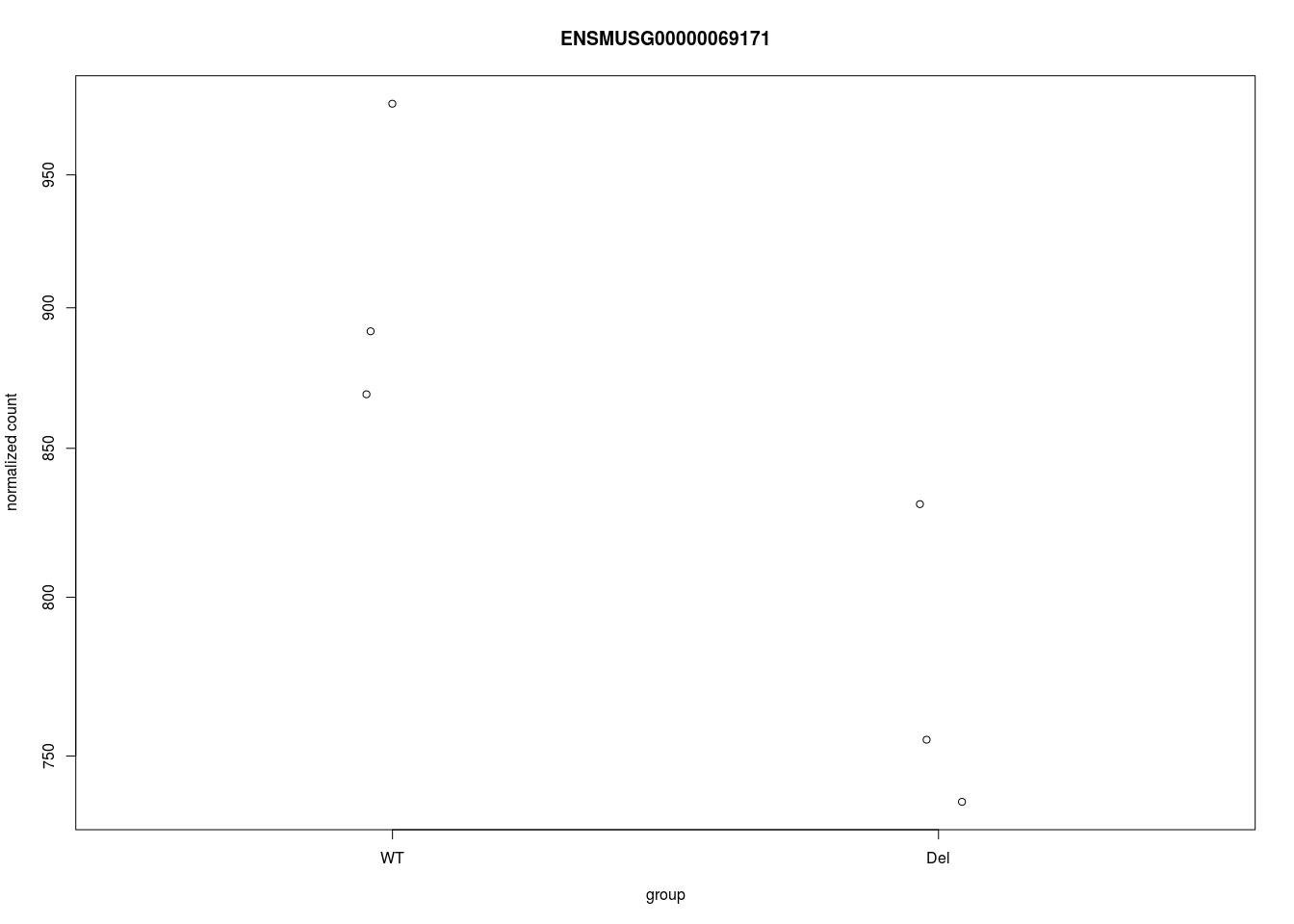# 11 Gene ontology enrichment analysis

We can now try characterize the identified differentially expressed genes a bit better by performing an GO enrichment analysis. Essentially the gene ontology () is a hierarchically organized collection of functional gene sets. For a nice introduction to the GO see

The function genefinder from the r Biocpkg("genefilter") package will be used to find background genes that are similar in expression to the differentially expressed genes. The function tries to identify 10 genes for each DE–gene that match its expression strength.

We then check whether the background has roughly the same distribution of average expression strength as the foreground by plotting the densities.

We do this in order not to select a biased background since the gene set testing is performed by a simple Fisher test on a 2x2 table, which uses only the status of a gene, i.e. whether it is differentially expressed or not and not its fold–change or absolute expression.

Note that the chance of a gene being identified as DE will most probably depend its gene expression for RNA–Seq data (potentially also its gene length etc.). Thus it is important to find a matching background. Our the testing approach here is very similar to web tools like DAVID, however, we explicitly model the background here.

We first get average gene expressions for each of the genes and then find non–DE genes that show a similar expression as the DE–genes. These genes are then our background.

overallBaseMean <- as.matrix(DESeq2Res[, "baseMean", drop = F])

sig_idx <- match(anSig$ENSEMBL, rownames(overallBaseMean)) backG <- c() for(i in sig_idx){ ind <- genefinder(overallBaseMean, i, 10, method = "manhattan")[]$indices
backG <- c(backG, ind)

}

backG <- unique(backG)
backG <- rownames(overallBaseMean)[backG]

We now remove DE genes from background and the get the total number of genes in the background.

backG <- setdiff(backG,  anSig$ENSEMBL) length(backG) ##  2437 Plotting the density of the average expressions, shows that the background matching has worked reasonably well.  multidensity( list( all= log2(DESeq2Res[,"baseMean"]) , foreground =log2(DESeq2Res[anSig$ENSEMBL, "baseMean"]),
background =log2(DESeq2Res[backG, "baseMean"])),
xlab="log2 mean normalized counts", main = "Matching for enrichment analysis")